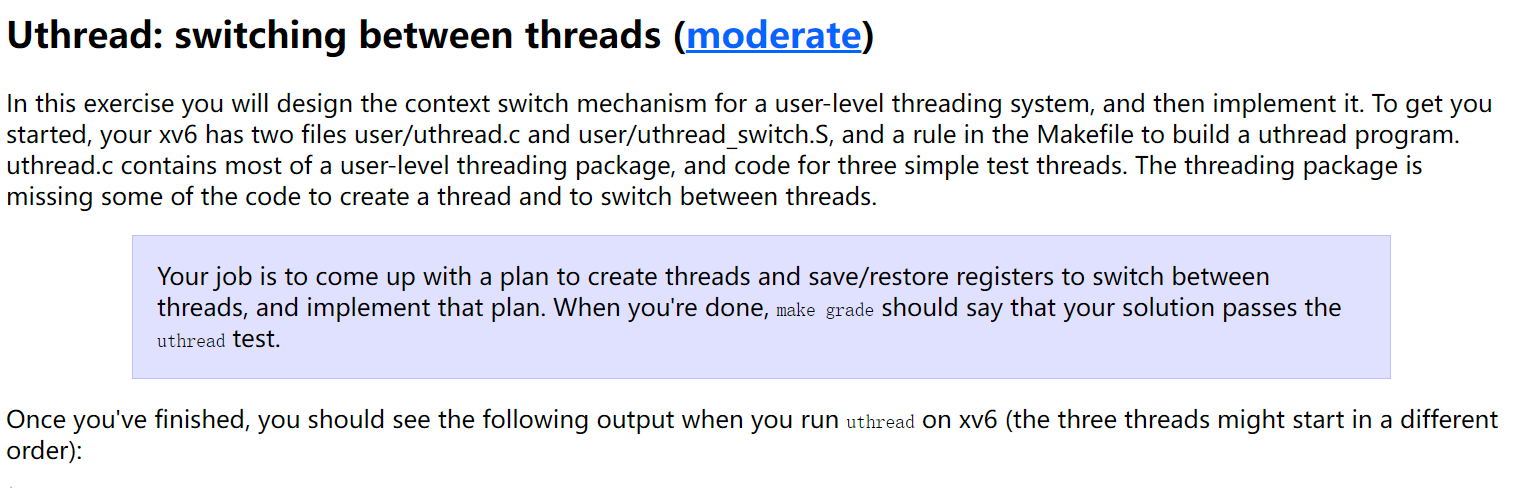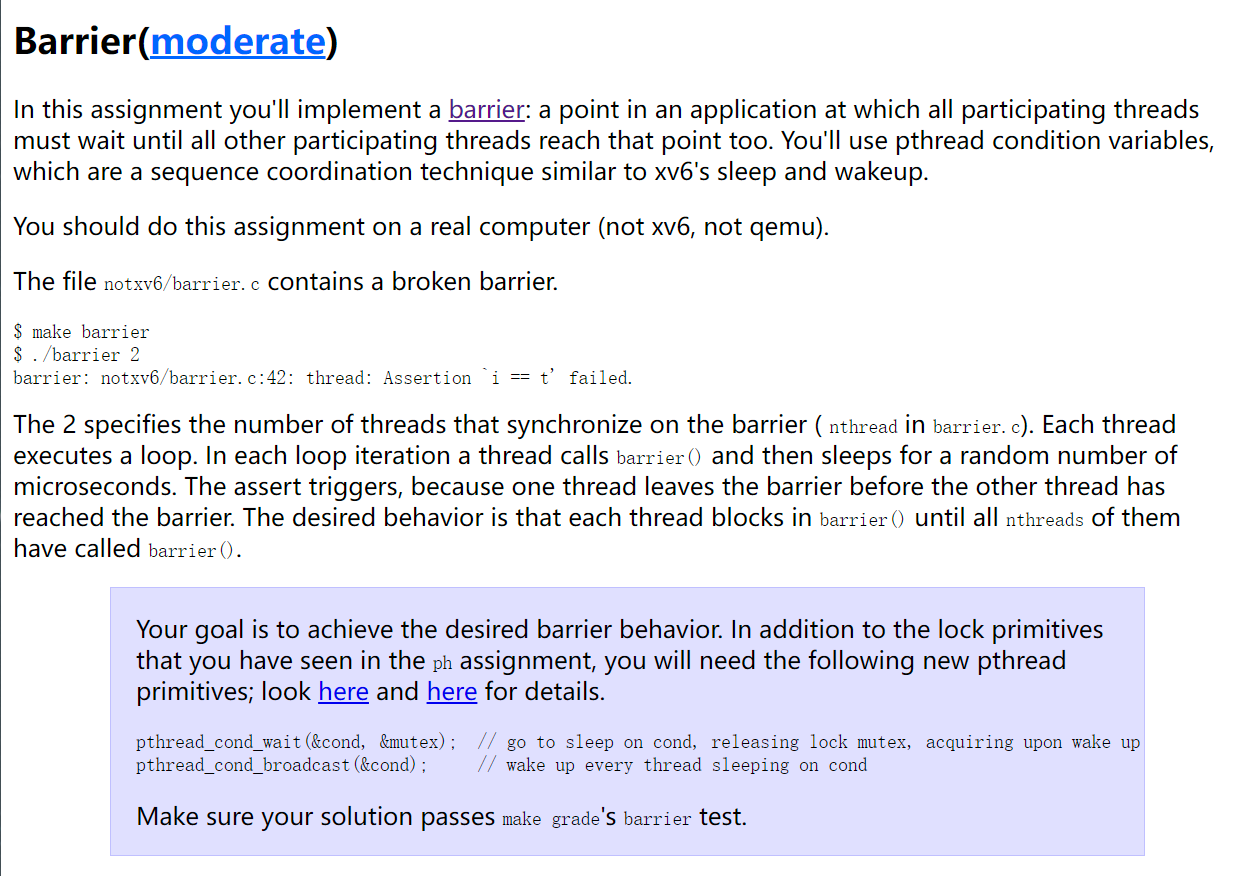upd@2022/9/14：最近把实验的代码放到 github 上了，如果需要参考可以查看这里：

https://github.com/ttzytt/xv6-riscv• thread_switch()： 这个函数和内核中的 swtch() 完全一样，用于切换处理器的上下文。和内核中相同（参考这篇文章），因为执行这个函数的过程是一个正常的函数调用，所以我们不需要保存和交换调用者保存的寄存器。
• thread_create() ：这个函数是用于创建新的用户线程的。参考内核态多线程的实现。我们调用 swtch() 后，决定跳转位置的是 ra 寄存器，决定恢复出来的被调用者保存寄存器的是 sp 寄存器。所以，在这个函数中，我们应该合理的设置 ra 寄存器，使得第一次执行用户函数时，是这个函数的第一条语句。
• thread_schedule()：参考内核中的实现，这个函数和内核中的 scheduler() 的作用相同。也就是在当前进程调用 yield() 后，找到一个 RUNNABLE 的进程，然后执行这个进程。在 thread_schedule() 中，我们会需要调用 thread_switch() 来切换处理器的上下文。

struct Context{
uint64 ra;
uint64 sp;

// callee-saved
uint64 s0;
uint64 s1;
uint64 s2;
uint64 s3;
uint64 s4;
uint64 s5;
uint64 s6;
uint64 s7;
uint64 s8;
uint64 s9;
uint64 s10;
uint64 s11;
};

char       stack[STACK_SIZE]; /* the thread's stack */
int        state;             /* FREE, RUNNING, RUNNABLE */
struct Context ctx;
};

 .text

/*
* save the old thread's registers,
* restore the new thread's registers.
*/

// a0 是老的上下文，a1 是新的
sd ra, 0(a0)
sd sp, 8(a0)
sd s0, 16(a0)
sd s1, 24(a0)
sd s2, 32(a0)
sd s3, 40(a0)
sd s4, 48(a0)
sd s5, 56(a0)
sd s6, 64(a0)
sd s7, 72(a0)
sd s8, 80(a0)
sd s9, 88(a0)
sd s10, 96(a0)
sd s11, 104(a0)

ld ra, 0(a1)
ld sp, 8(a1)
ld s0, 16(a1)
ld s1, 24(a1)
ld s2, 32(a1)
ld s3, 40(a1)
ld s4, 48(a1)
ld s5, 56(a1)
ld s6, 64(a1)
ld s7, 72(a1)
ld s8, 80(a1)
ld s9, 88(a1)
ld s10, 96(a1)
ld s11, 104(a1)



t->ctx.ra = (uint64) func;

t->ctx.sp = (uint64) &t->stack + (STACK_SIZE - 1);

void
{

if (t->state == FREE) break;
}
t->state = RUNNABLE;
t->ctx.ra = (uint64) func;
t->ctx.sp = (uint64) &t->stack + (STACK_SIZE - 1);
}

t = current_thread;
current_thread = next_thread; // 当前线程变成下一个线程了

thread_switch((uint64) &t->ctx, (uint64) &next_thread->ctx);

void
{

/* Find another runnable thread. */
for(int i = 0; i < MAX_THREAD; i++){
if(t->state == RUNNABLE) {
break;
}
t = t + 1;
}

exit(-1);
}

*/
} else
}

static void
insert(int key, int value, struct entry **p, struct entry *n)
{
struct entry *e = malloc(sizeof(struct entry));
e->key = key;
e->value = value;
e->next = n;
*p = e; // 把 p table[i] 的起始点改成 e
}

static
void put(int key, int value)
{
// is the key already present?
struct entry *e = 0;
for (e = table[i]; e != 0; e = e->next) {
if (e->key == key)
break;
}
if(e){
// update the existing key.
e->value = value;
} else {
// the new is new.
insert(key, value, &table[i], table[i]); // 在 table[i] 的最前面插入一个 key val 对
}
}

static struct entry*
get(int key)
{
int i = key % NBUCKET;
struct entry *e = 0;
for (e = table[i]; e != 0; e = e->next) {
if (e->key == key) break;
}
return e;
}

t1 先检查了链表中不存在 k1，于是准备调用 insert() 在链表前插入键值对。

pthread_mutex_t bkt_lock[NBUCKET];

static
void put(int key, int value)
{
int i = key % NBUCKET;

// is the key already present?
struct entry *e = 0;
for (e = table[i]; e != 0; e = e->next) {
if (e->key == key)
break;
}
if(e){
// update the existing key.
e->value = value;
} else {
// the new is new.
insert(key, value, &table[i], table[i]); // 在 table[i] 的最前面插入一个键值对
}
}

static struct entry*
get(int key)
{
int i = key % NBUCKET;

struct entry *e = 0;
for (e = table[i]; e != 0; e = e->next) {
if (e->key == key) break;
}
return e;
}

## Barrier2. 把线程放入等待条件的线程列表上
3. 阻塞线程（直到别的线程发送信号）。

2. 被唤醒的线程中，pthread_cond_wait() 会返回。
3. mutex 再次被锁住

lock(x_lock) // 拿到保护 x 的锁
if (x 满足条件){
unlock(x_lock); // 释放保护 x 的锁
lock(x_lock); // dosomething 可能会更改 x
dosomething();
}
unlock();

struct barrier {
int nthread;      // Number of threads that have reached this round of the barrier
int round;     // Barrier round
} bstate;

static void
barrier()
{
//
// Block until all threads have called barrier() and
// then increment bstate.round.
//
// 如果没有全部到达 barrier 的位置，就等待
// 在收到信号之前，这里是阻塞的
}else{ // 如果这是最后一个线程。
bstate.round++;
}
}